﻿ Java Polymorphism - Shape Class with Circle, Rectangle, and Triangle Subclasses

# Java Polymorphism Programming - Shape Class with Circle, Rectangle, and Triangle Subclasses for Area Calculation

## Java Polymorphism: Exercise-3 with Solution

Write a Java program to create a base class Shape with a method called calculateArea(). Create three subclasses: Circle, Rectangle, and Triangle. Override the calculateArea() method in each subclass to calculate and return the shape's area.

In the given exercise, here is a simple diagram illustrating polymorphism implementation: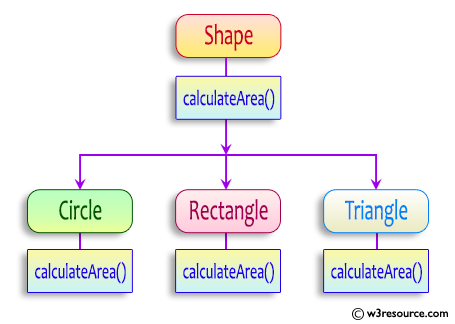In the above diagram, the Shape class is the base class, and it has a single method calculateArea(). The Circle, Rectangle, and Triangle classes inherit from the Shape class and override the calculateArea() method.

This diagram visually represents the class structure and the polymorphism relationship between the classes involved in the implementation.

Sample Solution:

Java Code:

``````// Shape.java
// Base class Shape
public class Shape {
public double calculateArea() {
return 0; // Default implementation returns 0
}
}
```
```
``````// Circle.java
// Subclass Circle
public class Circle extends Shape {

}

@Override
public double calculateArea() {
}
}
``````
``````// Rectangle.java
// Subclass Rectangle
public class Rectangle extends Shape {
private double width;
private double height;

public Rectangle(double width, double height) {
this.width = width;
this.height = height;
}

@Override
public double calculateArea() {
return width * height; // Calculate area of rectangle
}
}
``````
``````// Triangle.java
// Subclass Triangle
public class Triangle extends Shape {
private double base;
private double height;

public Triangle(double base, double height) {
this.base = base;
this.height = height;
}

@Override
public double calculateArea() {
return 0.5 * base * height; // Calculate area of triangle
}
}
``````
``````// Main.java
// Main class
public class Main {
public static void main(String[] args) {
Circle circle = new Circle(4);
System.out.println("Area of Circle: " + circle.calculateArea());

Rectangle rectangle = new Rectangle(12, 34);
System.out.println("\nArea of Rectangle: " + rectangle.calculateArea());

Triangle triangle = new Triangle(5, 9);
System.out.println("\nArea of Triangle: " + triangle.calculateArea());
}
}
``````

Sample Output:

```Area of Circle: 50.26548245743669

Area of Rectangle: 408.0

Area of Triangle: 22.5
```

Flowchart: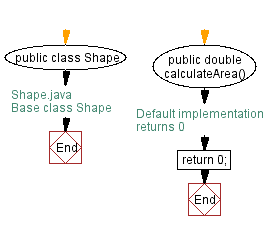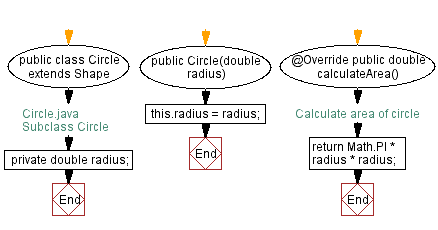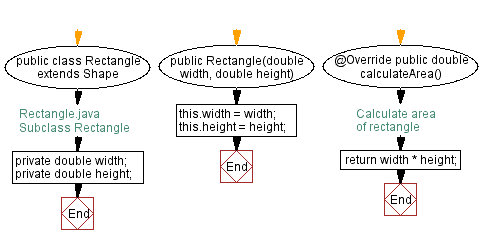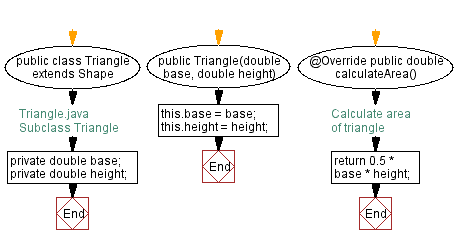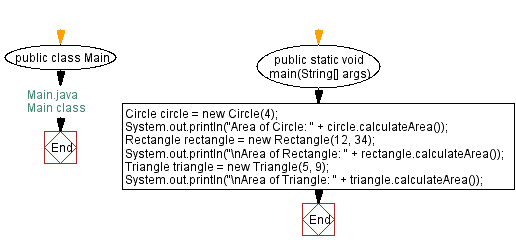Java Code Editor:

What is the difficulty level of this exercise?

﻿

## Java: Tips of the Day

How do I remove repeated elements from ArrayList?

If you don't want duplicates in a Collection, you should consider why you're using a Collection that allows duplicates. The easiest way to remove repeated elements is to add the contents to a Set (which will not allow duplicates) and then add the Set back to the ArrayList:

```Set<String> set = new HashSet<>(yourList);
yourList.clear();